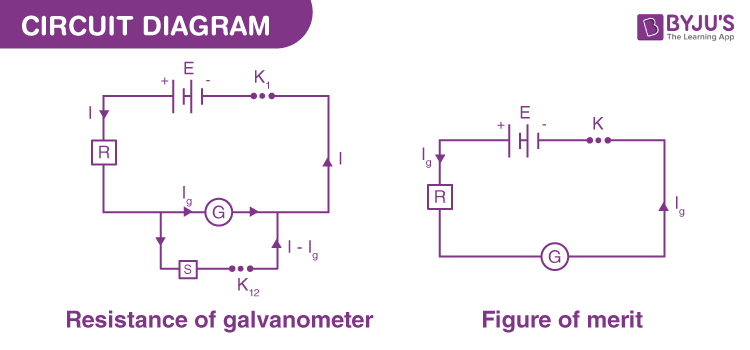# To Determine The Resistance Of A Galvanometer By A Half-deflection Method And To Find Its Figure Of Merit

## Aim

To determine the resistance of a galvanometer by a half-deflection method and to find its figure of merit.

## Apparatus/Materials Required

• A battery
• A Weston type galvanometer
• Two one-way keys
• Rheostat
• Screw gauge
• Metre-scale
• Ammeter
• Connecting wires
• A piece of sandpaper
• Two resistance boxes

## Circuit Diagram## Procedure

1. Connect the circuit as shown in the figure.
2. Make sure that all the plugs in the resistance boxes are tight
3. Eliminate the high resistance from the resistance box and insert plug key K1 only.
4. Adjust the value of R to make the deflection maximum both in number and within the scale.
5. Note the deflection θ.
6. Now insert the key K2 without changing the value of R. Adjust the value of S such that the value of the deflection reduces to half of θ, i.e. θ/2
7. Note down the value of resistance S.
8. Repeat steps 4 to 7 three times and obtains different values of R for different values of S.
9. Find the value of the E.M.F of one of the cells with the help of a voltmeter.

## Observations and Calculations

 S.No (1) Resistance R (2) Deflection in the Galvanometer θ (3) Shunt Resistance S (4) Half Deflection θ/2 (5) Galvanometer Resistance $$\begin{array}{l}G=\frac{RS}{R-S}\end{array}$$ (6) 1 2 3

Table for the resistance of the galvanometer by half deflection method

 S.No (1) Number of cells (Battery Eliminator) (2) e.m.f of the cells or the reading of the battery eliminator E(V) (3) Resistance from R.B. R (ohm) (4) Deflection θ (div) (5) Figure of merit $$\begin{array}{l}k=\frac{E}{(R+G)\Theta }\end{array}$$ (6) 1. 2. 3. 4.

The number of divisions in the galvanometer scale, n = _____.

1. Calculation for G
• Using the formula
$$\begin{array}{l}G=\frac{RS}{R-S}\end{array}$$
, calculate G and note it down in column 6 of Table 1.
• Take the mean of the value of G from column 6 of Table 1.
2. The calculation for k
• Using the formula
$$\begin{array}{l}k=\frac{E}{(R+G)\Theta }\end{array}$$
,
calculate the value of k and write it in column 6 of Table 2.
• Take the mean value of k and record it in column 2 of Table 2.

## Result

1. The resistance of the given galvanometer = _____ Ω.
2. The figure of merit of a given galvanometer = _____ A/dn.

## Precautions

1. All the plugs in the resistance box should be tight.
2. The e.m.f of the cell or battery should be constant.
3. High resistance from the resistance box should be introduced in the circuit otherwise excessive current will flow through the ammeter and it can be damaged.

## Viva Voce

1. What is a Galvanometer?
2. Ans: A galvanometer is a device that is used to detect feeble electric currents in the circuit.

3. Define the figure of merit of a galvanometer.
4. Ans: The figure of merit of a galvanometer is the quantity of current required to produce a deflection of one division in the galvanometer. It has a unit ampere per division and it is represented by K.

5. Why is the method called the half deflection method?
6. Ans: It is called so because the deflection is made half by using a shunt resistance S.

7. Under what condition is the resistance of a galvanometer (E1) equal to the shunt resistance S?
8. Ans: E1 = S, only when series resistance R is high.

Stay tuned with Byju’s to get the latest notification on CBSE along with the CBSE syllabus, sample papers, marking scheme and more.